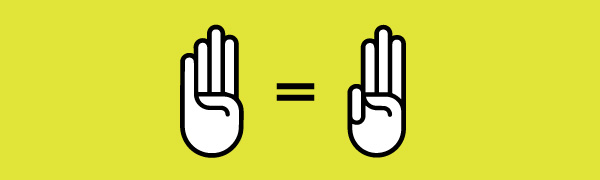Four equals to three

Algebra Level 2Kimmie wants to prove that $4=3$.

She starts off with the equation $a-b=c$ which is equivalent to

$\large 4a-3a-4b+3b = 4c-3c$

She moved the variables to obtain $4a-4b-4c = 3a-3b-3c$ and factorized it to

$\large 4(a-b-c) = 3(a-b-c)$

Finally, she cancelled both sides to obtain $4=3$.

What is the error in Kimmie's work?

×